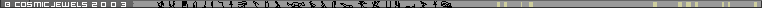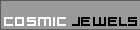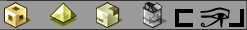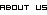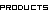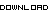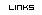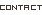PRODUCTsMerkabaSpiralThe golden meanCreationFlowerShefaMobiusEmeraldGenesisSeedTreeTorusGridViseca PiscesKaDune RingAlchemy PendantFour Winds RingLabyrinth PendantTHE GOLDEN MEAN SPIRAL:

 This crop circle shape known as the "Hackpen Hill Formation" appeared in an English wheat fielding 1999. It's structure represents two well known sacred geometry shapes: the golden mean (phi) spiral and fibonacci spiral. Both of these two spirals represent the Christ nsciousness grid of the human race that had been destroyed some 13000 years ago; this event caused future generations to perceive reality through low consciousness levels thus creating fear and chaos.
On the third dimension the grid looks like two spirals (if all the major ancient monuments upon this planet were to be connected we would discover the beginning of the spirals in Egypt). On the fourth dimension the grid resembles a combination of two Platonic Solids-the dodecahedron and the icosahedrons. the golden mean spiral can be found in  another constant proportion incorporated into the Pyramid of giza which is that of phi, the golden mean. Phi is a constant value which is even more mysterious and profound in its implications than pi. Like pi, phi is a number with no arithmetical solution. The decimals just keep on going into infinity without ever repeating themselves. The unique thing about this number is that it can be found incorporated in all known organic structures. From the bone structure of human beings to the seed pattern of a sunflower to the spiral of a sea shell, the phi proportion is there, underlying all biological structure, seeming to be a geometrical blueprint for life itself. Plato went so far as to call the phi ratio the "key to the physics of the cosmos."
Phi is calculated at 1.6180339+..., and while it cannot be worked out arithmetically, it can be easily obtained with a compass and straightedge. Two simple ways of finding the Golden Section geometrically are as follows: If you take two equal squares, side by side, (a 1x2 rectangle,) divide one of the squares in half, and with a compass, swing the diagonal down to the base of the other square, the point where the diagonal touches the base will be phi, or 1.6180339+, in relation to the side of the square, which is 1. ( This formula also describes exactly the rectangular floor of the King's Chamber.) The other simple method of determining the Golden Section is by dividing a line segment , AB, at a point, C, in such a way that the whole line is longer than the first part in the same proportion as the first part is longer than the remainder. AB/AC = AC/CB = 1.6180339. ( Notice the fractal and holographic nature of this ratio...) The phi ratio is found in the architecture of the Great Pyramid in the triangle formed by the height, half-base, and the apothem, or diagonal. In other words, the basic cross-section of the structure demonstrates the Golden Section. If the half-base is given a value of 1,this gives the value of phi for the apothem, and the square root of phi for the height. The Golden Section shows up again and again in Giza, and in much more baffling and terious ways. (Entire volumes have been written on the geometries involved in the pyramids' construction, we're just covering a few of the basics here.) There is a number sequence, known as the Fibonacci Sequence, which bears a special relationship to phi, and to the pyramids at Giza. First postulated by the medieval mathematician Leonardo Fibonacci, this sequence was used to describe the growth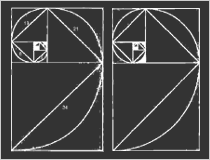patterns of plants. It goes: 1,1,2,3,5,8,13,21,34,55,89,144,233, etc. It is made by adding the last two numbers of the sequence to get the next one, like so: 1+1=2, 1+2=3, 2+3=5, 3+5=8, 5+8=13, etc. This sequence's relationship to phi is that if you divide one term of the sequence into the next one, he result begins to quickly approach the transcendental number 1.6180339+... (I won't make you do the math. Just watch...)
1/1=1 2\1=2 3\2=1.5 5\3=1.66 13/8=1.625
21/13=1.615 34\21=1.619 55\34=1.617 89\55=1.6181
The further you go down the sequence, the closer you get to phi. Of course, you can never actually get to it exactly, since it has no mathematical solution, it just keeps getting closer and closer, ad infinitum. Anyway, the sequence can be graphed out in the form of a spiral, called the Fibonacci Spiral. This spiral is almost identical to the graph of the logarithmic spiral of the phi ratio, known as the Golden Mean Spiral. (The difference is that the Fibonacci spiral is a whole-number interpretation of the arithmetically impossible Golden Mean spiral, which has no beginning or end. The Fibonacci spiral has a definite beginning.) click on image for better view.
Aerial photographs show that the pyramids at Giza are positioned in such a way that they fall on a line which accurately defines a Fibonacci spiral. The spiral passes exactly through the centers of each pyramid.

Source: Golden Spiral